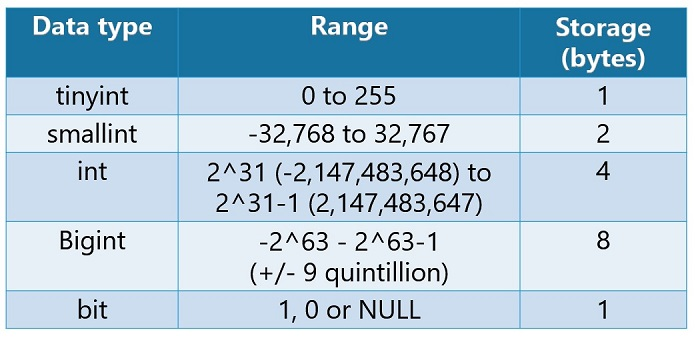# Integer Data TypesHere are some examples of the integer data types. When deciding on an integer for a column, variable, parameter, or expression it is a wise choice to choose the most efficient data type based off size and usage.

``````DECLARE @tiny as tinyint = 255
DECLARE @small as smallint = 32767
DECLARE @integer as int = 2147483647
DECLARE @big as bigint = 9223372036854775807``````

TINY INTEGER: The tiny integer data type allows for whole numbers between 0 and 255 and only uses 1 byte of data.

SMALL INTEGER: The small integer data type allows for a whole number between -32678 to a positive value of 32767 and will consume 2 bytes of data.

REGULAR INTEGER: The regular integer data type allows for a whole number between -2147483648 to a positive value 2147483647 and will consume 4 bytes of data.

BIG INTEGER: The big integer data type allows for a whole number between -9 quintillion to a value of positive 9 quintillion and will consume 8 bytes of data.

``````SELECT @tiny as Tiny_Integer,
@small as Small_Integer,
@integer as Integer,
@big as Big_Integer``````

#### Be the first to commenton "Integer Data Types"

This site uses Akismet to reduce spam. Learn how your comment data is processed.